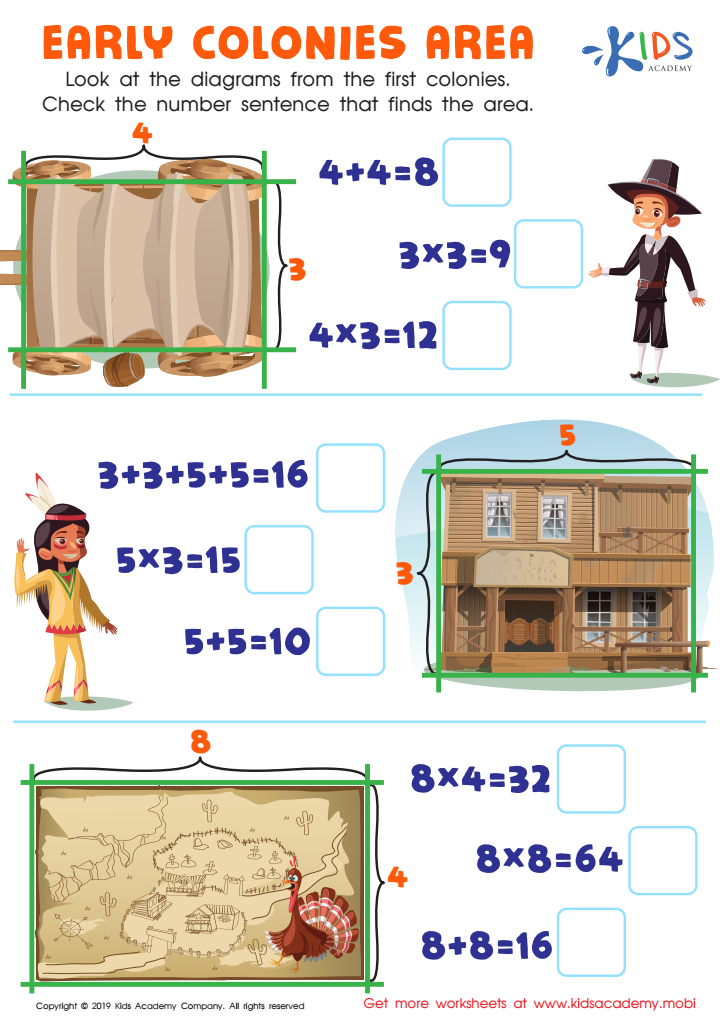43 filtered results

Clear all filters
Favorites
Online only

43 filtered results

Difficulty Level

Standards

Introducing our comprehensive Easy Math worksheets with answer key, designed specifically for Grade 3 learners. These worksheets are the perfect resource for reinforcing fundamental math skills in a fun and engaging way. Packed with a variety of exercises, students will practice addition, subtraction, multiplication, and division, as well as fractions, geometry, and measurement. With step-by-step instructions and clear visuals, these worksheets provide ample opportunities for students to grasp key concepts independently. The accompanying answer key ensures quick and accurate self-assessment, promoting confidence and progress. Equip your Grade 3 students with the essential math skills they need to excel with our Easy Math worksheets.

Favorites
Online only
• Math
• Easy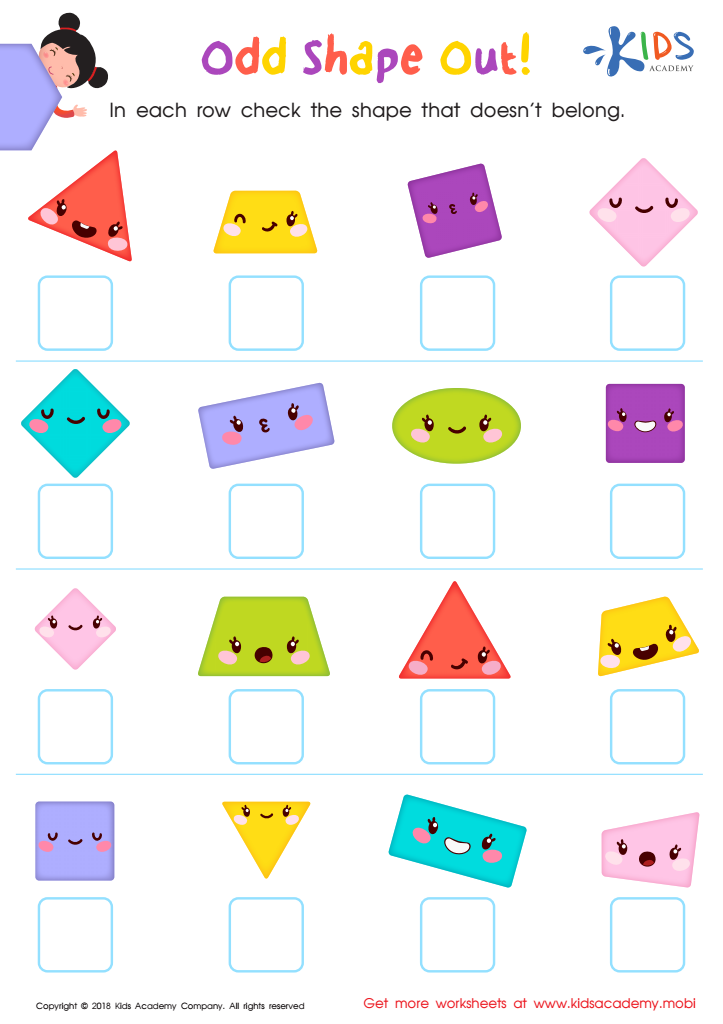Odd Shape Out Worksheet for Grade 3
Time to test your child's shape knowledge! With this worksheet, help them find the odd shape out in each row and check the box under it. Print out the pdf and look through it with them – each row has four brightly colored shapes. See if they can spot any differences among them and then check the box below the shape that does not belong.
Odd Shape Out Worksheet for Grade 3
Worksheet
Odd Shape Out Worksheet for Grade 3
Can your child draw and correctly identify different shapes? Now is the time to see …
Math
Worksheet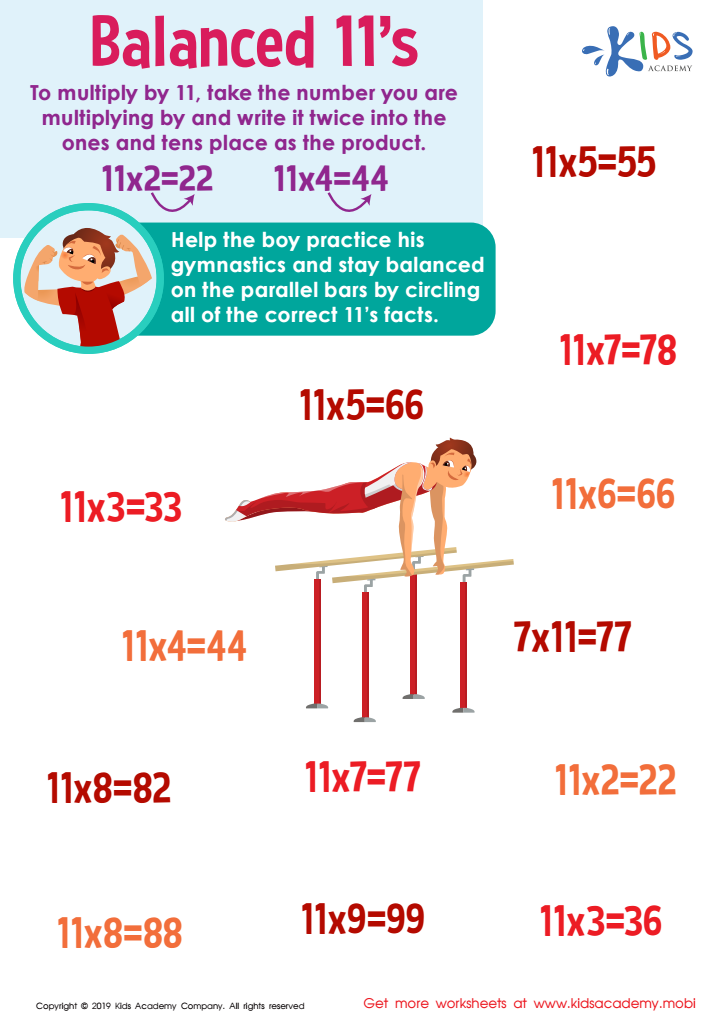Balanced 11s Worksheet
Explain to your kids that there are tricks for solving complex multiplication problems. Read the worksheet explaining how to multiply by 11 and have them practice with the boy in the picture. Show them how to circle the correct 11's facts for an easier understanding. This will help build better multiplication skills and understanding of figures.
Balanced 11s Worksheet
Worksheet
Balanced 11s Worksheet
Multiplication requires some pretty good skills, and a good understanding of figures on your child’s …
Math
Worksheet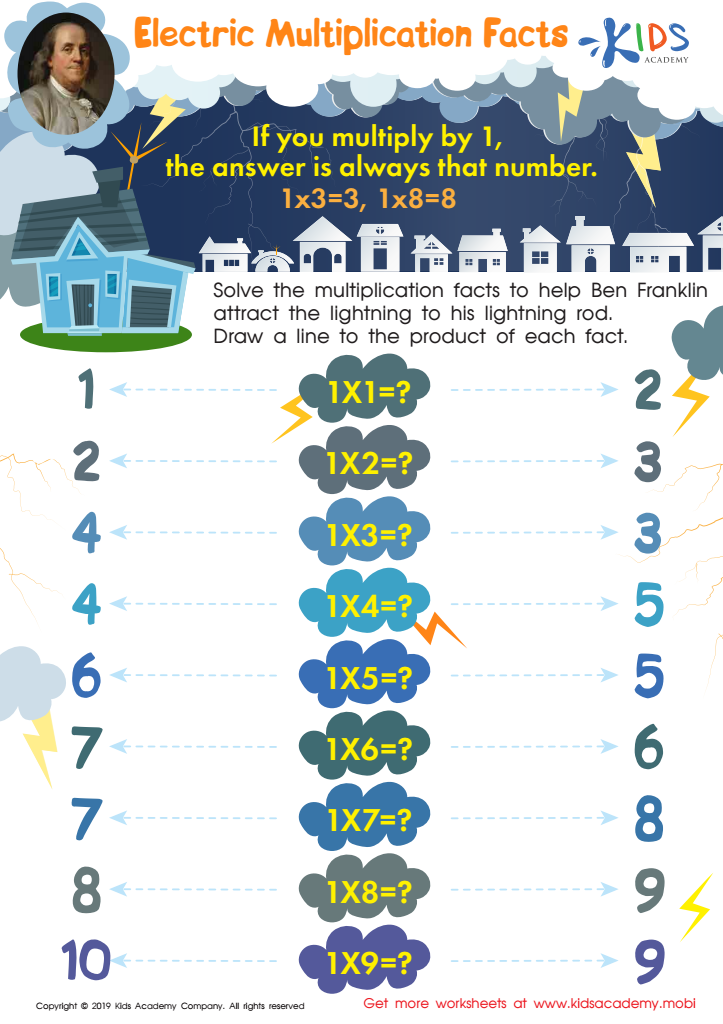Electric Multiplication Facts Worksheet
Assisting your kids with math homework has no limits. After mastering addition and subtraction, the next step is multiplication. Initially, it can be intimidating. However, with the right tips and worksheets like this one, they'll see it's not so tricky. Show them how multiplying by 1 keeps the number the same. Use the tracing sheet to help them work through multiplication facts.
Electric Multiplication Facts Worksheet
Worksheet
Electric Multiplication Facts Worksheet
There is really no end to helping your kids with their mathematics assignments. If they …
Math
Worksheet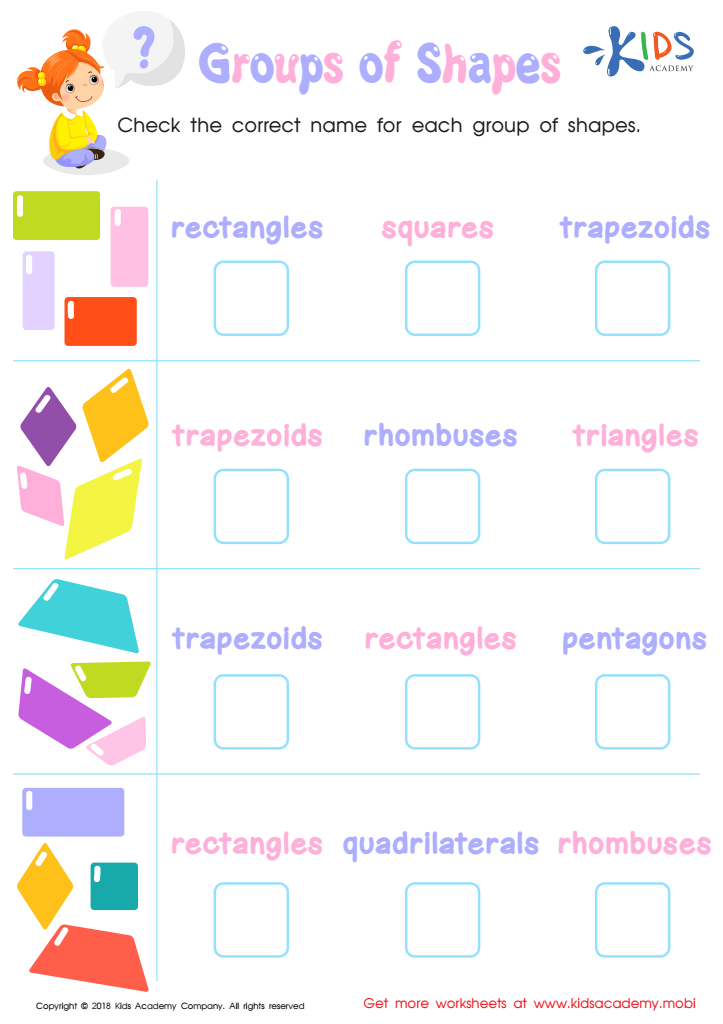Groups of Shapes Worksheet
Can your child identify and name shapes? Help them learn the unique features of each and have them check the correct name for each group in the colorful pictures on this worksheet.
Groups of Shapes Worksheet
Worksheet
Groups of Shapes Worksheet
How well can your child identify and name the different shapes? Shapes are each unique …
Math
Worksheet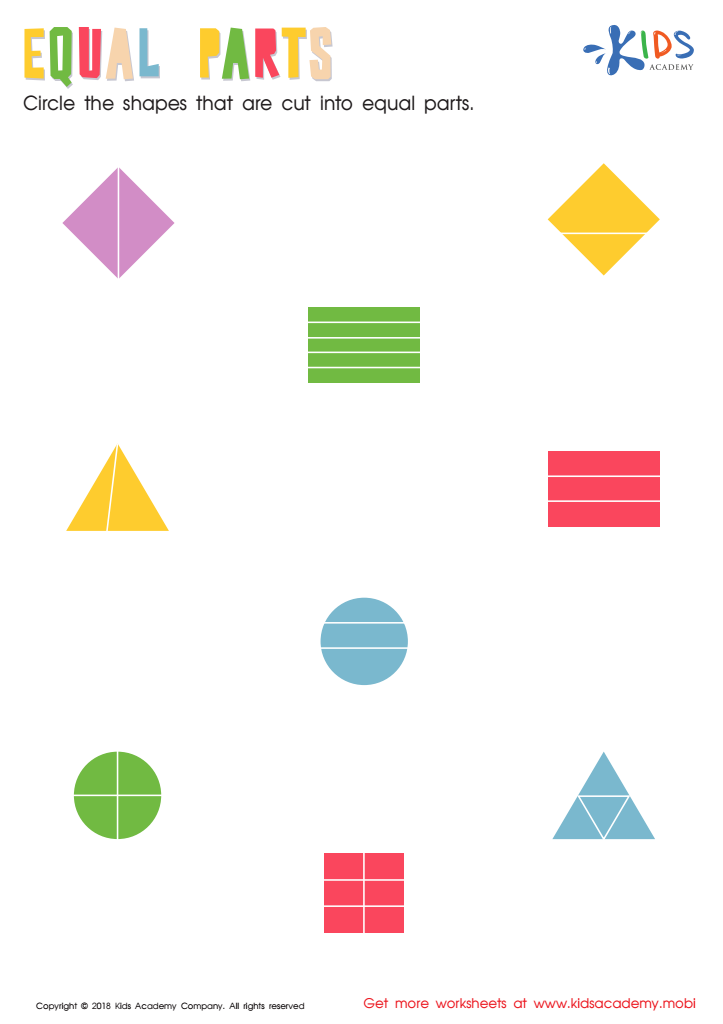Equal Parts: Shapes Worksheet
Test your child's ability to cut shapes into equal parts with this colorful printout. It includes rectangles, kites, circles, squares, and triangles - your child must circle the shapes that are split evenly. A simple task, but it will show if they understand the concept.
Equal Parts: Shapes Worksheet
Worksheet
Equal Parts: Shapes Worksheet
This equal parts worksheet is simple enough; your child must circle the shapes that are …
Math
Worksheet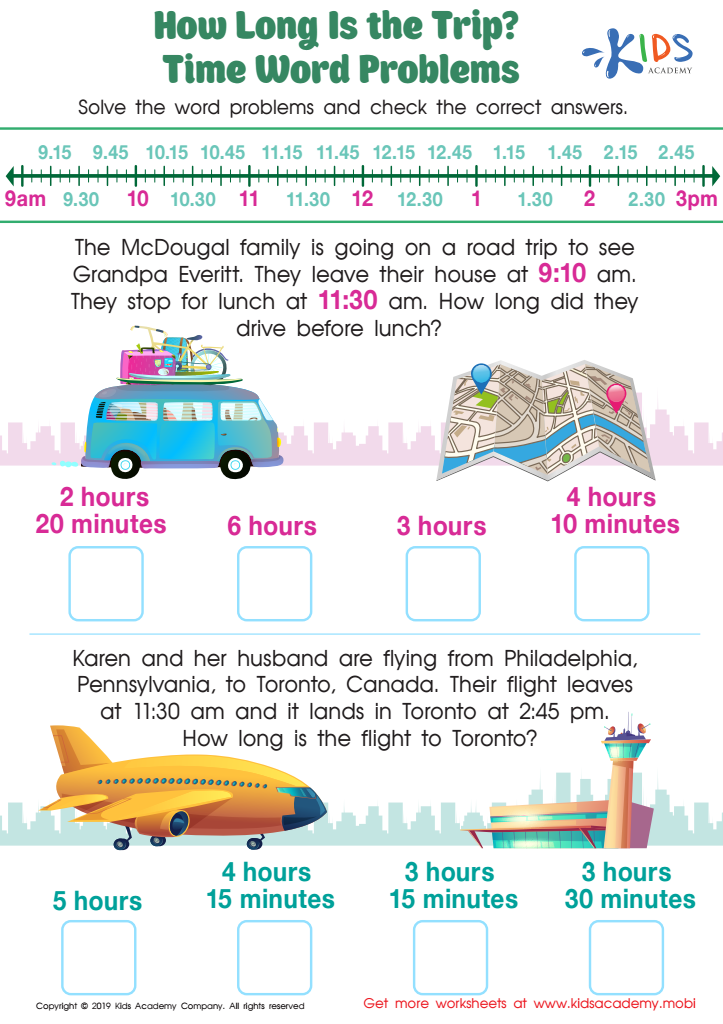How long is the Trip? Time Word Problems Worksheet
Get your kids in the mood for learning with fun questions about trips. Point at each picture and ask them to identify the mode of transport. Read the word problems, help them solve and check the answers.
How long is the Trip? Time Word Problems Worksheet
Worksheet
How long is the Trip? Time Word Problems Worksheet
Do your kids look forward to trips? What is their favorite trip which they can …